abhi270502/python-pandas-data-analysis - Jovian
Learn data science and machine learning by building real-world projects on Jovian

Analyzing Tabular Data using Python and PandasThis tutorial series is a beginner-friendly introduction to programming and data analysis using the Python programming language. These tutorials take a practical and coding-focused approach. The best way to learn the material is to execute the code and experiment with it yourself. Check out the full series here:

This tutorial covers the following topics:

• Reading a CSV file into a Pandas data frame
• Retrieving data from Pandas data frames
• Querying, soring, and analyzing data
• Merging, grouping, and aggregation of data
• Extracting useful information from dates
• Basic plotting using line and bar charts
• Writing data frames to CSV files

How to run the code

This tutorial is an executable Jupyter notebook hosted on Jovian. You can run this tutorial and experiment with the code examples in a couple of ways: using free online resources (recommended) or on your computer.

Option 1: Running using free online resources (1-click, recommended)

The easiest way to start executing the code is to click the Run button at the top of this page and select Run on Binder. You can also select "Run on Colab" or "Run on Kaggle", but you'll need to create an account on Google Colab or Kaggle to use these platforms.

Option 2: Running on your computer locally

To run the code on your computer locally, you'll need to set up Python, download the notebook and install the required libraries. We recommend using the Conda distribution of Python. Click the Run button at the top of this page, select the Run Locally option, and follow the instructions.

Jupyter Notebooks: This tutorial is a Jupyter notebook - a document made of cells. Each cell can contain code written in Python or explanations in plain English. You can execute code cells and view the results, e.g., numbers, messages, graphs, tables, files, etc., instantly within the notebook. Jupyter is a powerful platform for experimentation and analysis. Don't be afraid to mess around with the code & break things - you'll learn a lot by encountering and fixing errors. You can use the "Kernel > Restart & Clear Output" menu option to clear all outputs and start again from the top.

Reading a CSV file using Pandas

Pandas is a popular Python library used for working in tabular data (similar to the data stored in a spreadsheet). Pandas provides helper functions to read data from various file formats like CSV, Excel spreadsheets, HTML tables, JSON, SQL, and more. Let's download a file italy-covid-daywise.txt which contains day-wise Covid-19 data for Italy in the following format:

date,new_cases,new_deaths,new_tests
2020-04-21,2256.0,454.0,28095.0
2020-04-22,2729.0,534.0,44248.0
2020-04-23,3370.0,437.0,37083.0
2020-04-24,2646.0,464.0,95273.0
2020-04-25,3021.0,420.0,38676.0
2020-04-26,2357.0,415.0,24113.0
2020-04-27,2324.0,260.0,26678.0
2020-04-28,1739.0,333.0,37554.0
...

This format of storing data is known as comma-separated values or CSV.

CSVs: A comma-separated values (CSV) file is a delimited text file that uses a comma to separate values. Each line of the file is a data record. Each record consists of one or more fields, separated by commas. A CSV file typically stores tabular data (numbers and text) in plain text, in which case each line will have the same number of fields. (Wikipedia)

We'll download this file using the urlretrieve function from the urllib.request module.

#restart the kernel after installation
from urllib.request import urlretrieve
italy_covid_url = 'https://gist.githubusercontent.com/aakashns/f6a004fa20c84fec53262f9a8bfee775/raw/f309558b1cf5103424cef58e2ecb8704dcd4d74c/italy-covid-daywise.csv'

urlretrieve(italy_covid_url, 'italy-covid-daywise.csv')
('italy-covid-daywise.csv', <http.client.HTTPMessage at 0x7f87ec447b80>)

To read the file, we can use the read_csv method from Pandas. First, let's install the Pandas library.

We can now import the pandas module. As a convention, it is imported with the alias pd.

import pandas as pd

Data from the file is read and stored in a DataFrame object - one of the core data structures in Pandas for storing and working with tabular data. We typically use the _df suffix in the variable names for dataframes.

type(covid_df)
pandas.core.frame.DataFrame
covid_df

Here's what we can tell by looking at the dataframe:

• The file provides four day-wise counts for COVID-19 in Italy
• The metrics reported are new cases, deaths, and tests
• Data is provided for 248 days: from Dec 12, 2019, to Sep 3, 2020

Keep in mind that these are officially reported numbers. The actual number of cases & deaths may be higher, as not all cases are diagnosed.

We can view some basic information about the data frame using the .info method.

covid_df.info()
<class 'pandas.core.frame.DataFrame'> RangeIndex: 248 entries, 0 to 247 Data columns (total 4 columns): # Column Non-Null Count Dtype --- ------ -------------- ----- 0 date 248 non-null object 1 new_cases 248 non-null float64 2 new_deaths 248 non-null float64 3 new_tests 135 non-null float64 dtypes: float64(3), object(1) memory usage: 7.9+ KB

It appears that each column contains values of a specific data type. You can view statistical information for numerical columns (mean, standard deviation, minimum/maximum values, and the number of non-empty values) using the .describe method.

covid_df.describe()

The columns property contains the list of columns within the data frame.

covid_df.columns
Index(['date', 'new_cases', 'new_deaths', 'new_tests'], dtype='object')

You can also retrieve the number of rows and columns in the data frame using the .shape method.

covid_df.shape
(248, 4)

Here's a summary of the functions & methods we've looked at so far:

• pd.read_csv - Read data from a CSV file into a Pandas DataFrame object
• .info() - View basic infomation about rows, columns & data types
• .describe() - View statistical information about numeric columns
• .columns - Get the list of column names
• .shape - Get the number of rows & columns as a tuple

Whether you're running this Jupyter notebook online or on your computer, it's essential to save your work from time to time. You can continue working on a saved notebook later or share it with friends and colleagues to let them execute your code. Jovian offers an easy way of saving and sharing your Jupyter notebooks online.

# Install the library
import jovian
jovian.commit(project='python-pandas-data-analysis')
[jovian] Attempting to save notebook.. [jovian] Updating notebook "aakashns/python-pandas-data-analysis" on https://jovian.ai/ [jovian] Uploading notebook.. [jovian] Capturing environment.. [jovian] Committed successfully! https://jovian.ai/aakashns/python-pandas-data-analysis

The first time you run jovian.commit, you'll be asked to provide an API Key to securely upload the notebook to your Jovian account. You can get the API key from your Jovian profile page after logging in / signing up.

Retrieving data from a data frame

The first thing you might want to do is retrieve data from this data frame, e.g., the counts of a specific day or the list of values in a particular column. To do this, it might help to understand the internal representation of data in a data frame. Conceptually, you can think of a dataframe as a dictionary of lists: keys are column names, and values are lists/arrays containing data for the respective columns.

# Pandas format is simliar to this
covid_data_dict = {
'date':       ['2020-08-30', '2020-08-31', '2020-09-01', '2020-09-02', '2020-09-03'],
'new_cases':  [1444, 1365, 996, 975, 1326],
'new_deaths': [1, 4, 6, 8, 6],
'new_tests': [53541, 42583, 54395, None, None]
}

Representing data in the above format has a few benefits:

• All values in a column typically have the same type of value, so it's more efficient to store them in a single array.
• Retrieving the values for a particular row simply requires extracting the elements at a given index from each column array.
• The representation is more compact (column names are recorded only once) compared to other formats that use a dictionary for each row of data (see the example below).
# Pandas format is not similar to this
covid_data_list = [
{'date': '2020-08-30', 'new_cases': 1444, 'new_deaths': 1, 'new_tests': 53541},
{'date': '2020-08-31', 'new_cases': 1365, 'new_deaths': 4, 'new_tests': 42583},
{'date': '2020-09-01', 'new_cases': 996, 'new_deaths': 6, 'new_tests': 54395},
{'date': '2020-09-02', 'new_cases': 975, 'new_deaths': 8 },
{'date': '2020-09-03', 'new_cases': 1326, 'new_deaths': 6},
]

With the dictionary of lists analogy in mind, you can now guess how to retrieve data from a data frame. For example, we can get a list of values from a specific column using the [] indexing notation.

covid_data_dict['new_cases']
[1444, 1365, 996, 975, 1326]
covid_df['new_cases']
0         0.0
1         0.0
2         0.0
3         0.0
4         0.0
...
243    1444.0
244    1365.0
245     996.0
246     975.0
247    1326.0
Name: new_cases, Length: 248, dtype: float64

Each column is represented using a data structure called Series, which is essentially a numpy array with some extra methods and properties.

type(covid_df['new_cases'])
pandas.core.series.Series

Like arrays, you can retrieve a specific value with a series using the indexing notation [].

covid_df['new_cases']
975.0
covid_df['new_tests']
57640.0

Pandas also provides the .at method to retrieve the element at a specific row & column directly.

covid_df.at[246, 'new_cases']
975.0
covid_df.at[240, 'new_tests']
57640.0

Instead of using the indexing notation [], Pandas also allows accessing columns as properties of the dataframe using the . notation. However, this method only works for columns whose names do not contain spaces or special characters.

covid_df.new_cases
0         0.0
1         0.0
2         0.0
3         0.0
4         0.0
...
243    1444.0
244    1365.0
245     996.0
246     975.0
247    1326.0
Name: new_cases, Length: 248, dtype: float64

Further, you can also pass a list of columns within the indexing notation [] to access a subset of the data frame with just the given columns.

cases_df = covid_df[['date', 'new_cases']]
cases_df

The new data frame cases_df is simply a "view" of the original data frame covid_df. Both point to the same data in the computer's memory. Changing any values inside one of them will also change the respective values in the other. Sharing data between data frames makes data manipulation in Pandas blazing fast. You needn't worry about the overhead of copying thousands or millions of rows every time you want to create a new data frame by operating on an existing one.

Sometimes you might need a full copy of the data frame, in which case you can use the copy method.

covid_df_copy = covid_df.copy()

The data within covid_df_copy is completely separate from covid_df, and changing values inside one of them will not affect the other.

To access a specific row of data, Pandas provides the .loc method.

covid_df
covid_df.loc
date          2020-08-30
new_cases         1444.0
new_deaths           1.0
new_tests        53541.0
Name: 243, dtype: object

Each retrieved row is also a Series object.

type(covid_df.loc)
pandas.core.series.Series

We can use the .head and .tail methods to view the first or last few rows of data.

covid_df.tail(4)

Notice above that while the first few values in the new_cases and new_deaths columns are 0, the corresponding values within the new_tests column are NaN. That is because the CSV file does not contain any data for the new_tests column for specific dates (you can verify this by looking into the file). These values may be missing or unknown.

covid_df.at[0, 'new_tests']
nan
type(covid_df.at[0, 'new_tests'])
numpy.float64

The distinction between 0 and NaN is subtle but important. In this dataset, it represents that daily test numbers were not reported on specific dates. Italy started reporting daily tests on Apr 19, 2020. 93,5310 tests had already been conducted before Apr 19.

We can find the first index that doesn't contain a NaN value using a column's first_valid_index method.

covid_df.new_tests.first_valid_index()
111

Let's look at a few rows before and after this index to verify that the values change from NaN to actual numbers. We can do this by passing a range to loc.

covid_df.loc[108:113]

We can use the .sample method to retrieve a random sample of rows from the data frame.

covid_df.sample(10)

Notice that even though we have taken a random sample, each row's original index is preserved - this is a useful property of data frames.

Here's a summary of the functions & methods we looked at in this section:

• covid_df['new_cases'] - Retrieving columns as a Series using the column name
• new_cases - Retrieving values from a Series using an index
• covid_df.at[243, 'new_cases'] - Retrieving a single value from a data frame
• covid_df.copy() - Creating a deep copy of a data frame
• covid_df.loc - Retrieving a row or range of rows of data from the data frame
• head, tail, and sample - Retrieving multiple rows of data from the data frame
• covid_df.new_tests.first_valid_index - Finding the first non-empty index in a series

Let's save a snapshot of our notebook before continuing.

import jovian
jovian.commit()

Analyzing data from data frames

Q: What are the total number of reported cases and deaths related to Covid-19 in Italy?

Similar to Numpy arrays, a Pandas series supports the sum method to answer these questions.

total_cases = covid_df.new_cases.sum()
total_deaths = covid_df.new_deaths.sum()
print('The number of reported cases is {} and the number of reported deaths is {}.'.format(int(total_cases), int(total_deaths)))
The number of reported cases is 271515 and the number of reported deaths is 35497.

Q: What is the overall death rate (ratio of reported deaths to reported cases)?

death_rate = covid_df.new_deaths.sum() / covid_df.new_cases.sum()
print("The overall reported death rate in Italy is {:.2f} %.".format(death_rate*100))
The overall reported death rate in Italy is 13.07 %.

Q: What is the overall number of tests conducted? A total of 935310 tests were conducted before daily test numbers were reported.

initial_tests = 935310
total_tests = initial_tests + covid_df.new_tests.sum()
total_tests
5214766.0

Q: What fraction of tests returned a positive result?

positive_rate = total_cases / total_tests
print('{:.2f}% of tests in Italy led to a positive diagnosis.'.format(positive_rate*100))
5.21% of tests in Italy led to a positive diagnosis.

Let's save and commit our work before continuing.

import jovian
jovian.commit()

Querying and sorting rows

Let's say we want only want to look at the days which had more than 1000 reported cases. We can use a boolean expression to check which rows satisfy this criterion.

high_new_cases = covid_df.new_cases > 1000
high_new_cases
0      False
1      False
2      False
3      False
4      False
...
243     True
244     True
245    False
246    False
247     True
Name: new_cases, Length: 248, dtype: bool

The boolean expression returns a series containing True and False boolean values. You can use this series to select a subset of rows from the original dataframe, corresponding to the True values in the series.

covid_df[high_new_cases]

We can write this succinctly on a single line by passing the boolean expression as an index to the data frame.

high_cases_df = covid_df[covid_df.new_cases > 1000]
high_cases_df

The data frame contains 72 rows, but only the first & last five rows are displayed by default with Jupyter for brevity. We can change some display options to view all the rows.

from IPython.display import display
with pd.option_context('display.max_rows', 100):
display(covid_df[covid_df.new_cases > 1000])

We can also formulate more complex queries that involve multiple columns. As an example, let's try to determine the days when the ratio of cases reported to tests conducted is higher than the overall positive_rate.

positive_rate
0.05206657403227681
high_ratio_df = covid_df[covid_df.new_cases / covid_df.new_tests > positive_rate]
high_ratio_df

The result of performing an operation on two columns is a new series.

covid_df.new_cases / covid_df.new_tests
0           NaN
1           NaN
2           NaN
3           NaN
4           NaN
...
243    0.026970
244    0.032055
245    0.018311
246         NaN
247         NaN
Length: 248, dtype: float64

We can use this series to add a new column to the data frame.

covid_df['positive_rate'] = covid_df.new_cases / covid_df.new_tests
covid_df

However, keep in mind that sometimes it takes a few days to get the results for a test, so we can't compare the number of new cases with the number of tests conducted on the same day. Any inference based on this positive_rate column is likely to be incorrect. It's essential to watch out for such subtle relationships that are often not conveyed within the CSV file and require some external context. It's always a good idea to read through the documentation provided with the dataset or ask for more information.

For now, let's remove the positive_rate column using the drop method.

covid_df.drop(columns=['positive_rate'], inplace=True)

Can you figure the purpose of the inplace argument?

Sorting rows using column values

The rows can also be sorted by a specific column using .sort_values. Let's sort to identify the days with the highest number of cases, then chain it with the head method to list just the first ten results.

It looks like the last two weeks of March had the highest number of daily cases. Let's compare this to the days where the highest number of deaths were recorded.

It appears that daily deaths hit a peak just about a week after the peak in daily new cases.

Let's also look at the days with the least number of cases. We might expect to see the first few days of the year on this list.

It seems like the count of new cases on Jun 20, 2020, was -148, a negative number! Not something we might have expected, but that's the nature of real-world data. It could be a data entry error, or the government may have issued a correction to account for miscounting in the past. Can you dig through news articles online and figure out why the number was negative?

Let's look at some days before and after Jun 20, 2020.

covid_df.loc[169:175]

For now, let's assume this was indeed a data entry error. We can use one of the following approaches for dealing with the missing or faulty value:

1. Replace it with 0.
2. Replace it with the average of the entire column
3. Replace it with the average of the values on the previous & next date

Which approach you pick requires some context about the data and the problem. In this case, since we are dealing with data ordered by date, we can go ahead with the third approach.

You can use the .at method to modify a specific value within the dataframe.

covid_df.at[172, 'new_cases'] = (covid_df.at[171, 'new_cases'] + covid_df.at[173, 'new_cases'])/2

Here's a summary of the functions & methods we looked at in this section:

• covid_df.new_cases.sum() - Computing the sum of values in a column or series
• covid_df[covid_df.new_cases > 1000] - Querying a subset of rows satisfying the chosen criteria using boolean expressions
• df['pos_rate'] = df.new_cases/df.new_tests - Adding new columns by combining data from existing columns
• covid_df.drop('positive_rate') - Removing one or more columns from the data frame
• sort_values - Sorting the rows of a data frame using column values
• covid_df.at[172, 'new_cases'] = ... - Replacing a value within the data frame

Let's save and commit our work before continuing.

import jovian
jovian.commit()

Working with dates

While we've looked at overall numbers for the cases, tests, positive rate, etc., it would also be useful to study these numbers on a month-by-month basis. The date column might come in handy here, as Pandas provides many utilities for working with dates.

covid_df.date
0      2019-12-31
1      2020-01-01
2      2020-01-02
3      2020-01-03
4      2020-01-04
...
243    2020-08-30
244    2020-08-31
245    2020-09-01
246    2020-09-02
247    2020-09-03
Name: date, Length: 248, dtype: object

The data type of date is currently object, so Pandas does not know that this column is a date. We can convert it into a datetime column using the pd.to_datetime method.

covid_df['date'] = pd.to_datetime(covid_df.date)
covid_df['date']
0     2019-12-31
1     2020-01-01
2     2020-01-02
3     2020-01-03
4     2020-01-04
...
243   2020-08-30
244   2020-08-31
245   2020-09-01
246   2020-09-02
247   2020-09-03
Name: date, Length: 248, dtype: datetime64[ns]

You can see that it now has the datatype datetime64. We can now extract different parts of the data into separate columns, using the DatetimeIndex class (view docs).

covid_df['year'] = pd.DatetimeIndex(covid_df.date).year
covid_df['month'] = pd.DatetimeIndex(covid_df.date).month
covid_df['day'] = pd.DatetimeIndex(covid_df.date).day
covid_df['weekday'] = pd.DatetimeIndex(covid_df.date).weekday
covid_df

Let's check the overall metrics for May. We can query the rows for May, choose a subset of columns, and use the sum method to aggregate each selected column's values.

# Query the rows for May
covid_df_may = covid_df[covid_df.month == 5]

# Extract the subset of columns to be aggregated
covid_df_may_metrics = covid_df_may[['new_cases', 'new_deaths', 'new_tests']]

# Get the column-wise sum
covid_may_totals = covid_df_may_metrics.sum()
covid_may_totals
new_cases       29073.0
new_deaths       5658.0
new_tests     1078720.0
dtype: float64
type(covid_may_totals)
pandas.core.series.Series

We can also combine the above operations into a single statement.

covid_df[covid_df.month == 5][['new_cases', 'new_deaths', 'new_tests']].sum()
new_cases       29073.0
new_deaths       5658.0
new_tests     1078720.0
dtype: float64

As another example, let's check if the number of cases reported on Sundays is higher than the average number of cases reported every day. This time, we might want to aggregate columns using the .mean method.

# Overall average
covid_df.new_cases.mean()
1096.6149193548388
# Average for Sundays
covid_df[covid_df.weekday == 6].new_cases.mean()
1247.2571428571428

It seems like more cases were reported on Sundays compared to other days.

Let's save and commit our work before continuing.

import jovian
jovian.commit()

Grouping and aggregation

As a next step, we might want to summarize the day-wise data and create a new dataframe with month-wise data. We can use the groupby function to create a group for each month, select the columns we wish to aggregate, and aggregate them using the sum method.

covid_month_df = covid_df.groupby('month')[['new_cases', 'new_deaths', 'new_tests']].sum()
covid_month_df

The result is a new data frame that uses unique values from the column passed to groupby as the index. Grouping and aggregation is a powerful method for progressively summarizing data into smaller data frames.

Instead of aggregating by sum, you can also aggregate by other measures like mean. Let's compute the average number of daily new cases, deaths, and tests for each month.

covid_month_mean_df = covid_df.groupby('month')[['new_cases', 'new_deaths', 'new_tests']].mean()
covid_month_mean_df

Apart from grouping, another form of aggregation is the running or cumulative sum of cases, tests, or death up to each row's date. We can use the cumsum method to compute the cumulative sum of a column as a new series. Let's add three new columns: total_cases, total_deaths, and total_tests.

covid_df['total_cases'] = covid_df.new_cases.cumsum()
covid_df['total_deaths'] = covid_df.new_deaths.cumsum()
covid_df['total_tests'] = covid_df.new_tests.cumsum() + initial_tests

We've also included the initial test count in total_test to account for tests conducted before daily reporting was started.

covid_df

Notice how the NaN values in the total_tests column remain unaffected.

Merging data from multiple sources

To determine other metrics like test per million, cases per million, etc., we require some more information about the country, viz. its population. Let's download another file locations.csv that contains health-related information for many countries, including Italy.

urlretrieve('https://gist.githubusercontent.com/aakashns/8684589ef4f266116cdce023377fc9c8/raw/99ce3826b2a9d1e6d0bde7e9e559fc8b6e9ac88b/locations.csv',
'locations.csv')
('locations.csv', <http.client.HTTPMessage at 0x7f87c57a41f0>)
locations_df
locations_df[locations_df.location == "Italy"]

We can merge this data into our existing data frame by adding more columns. However, to merge two data frames, we need at least one common column. Let's insert a location column in the covid_df dataframe with all values set to "Italy".

covid_df['location'] = "Italy"
covid_df

We can now add the columns from locations_df into covid_df using the .merge method.

merged_df = covid_df.merge(locations_df, on="location")
merged_df

The location data for Italy is appended to each row within covid_df. If the covid_df data frame contained data for multiple locations, then the respective country's location data would be appended for each row.

We can now calculate metrics like cases per million, deaths per million, and tests per million.

merged_df['cases_per_million'] = merged_df.total_cases * 1e6 / merged_df.population
merged_df['deaths_per_million'] = merged_df.total_deaths * 1e6 / merged_df.population
merged_df['tests_per_million'] = merged_df.total_tests * 1e6 / merged_df.population
merged_df

Let's save and commit our work before continuing.

import jovian
jovian.commit()

Writing data back to files

After completing your analysis and adding new columns, you should write the results back to a file. Otherwise, the data will be lost when the Jupyter notebook shuts down. Before writing to file, let us first create a data frame containing just the columns we wish to record.

result_df = merged_df[['date',
'new_cases',
'total_cases',
'new_deaths',
'total_deaths',
'new_tests',
'total_tests',
'cases_per_million',
'deaths_per_million',
'tests_per_million']]
result_df

To write the data from the data frame into a file, we can use the to_csv function.

result_df.to_csv('results.csv', index=None)

The to_csv function also includes an additional column for storing the index of the dataframe by default. We pass index=None to turn off this behavior. You can now verify that the results.csv is created and contains data from the data frame in CSV format:

date,new_cases,total_cases,new_deaths,total_deaths,new_tests,total_tests,cases_per_million,deaths_per_million,tests_per_million
2020-02-27,78.0,400.0,1.0,12.0,,,6.61574439992122,0.1984723319976366,
2020-02-28,250.0,650.0,5.0,17.0,,,10.750584649871982,0.28116913699665186,
2020-02-29,238.0,888.0,4.0,21.0,,,14.686952567825108,0.34732658099586405,
2020-03-01,240.0,1128.0,8.0,29.0,,,18.656399207777838,0.47964146899428844,
2020-03-02,561.0,1689.0,6.0,35.0,,,27.93498072866735,0.5788776349931067,
2020-03-03,347.0,2036.0,17.0,52.0,,,33.67413899559901,0.8600467719897585,
...

You can attach the results.csv file to our notebook while uploading it to Jovian using the outputs argument to jovian.commit.

import jovian
jovian.commit(outputs=['results.csv'])
[jovian] Attempting to save notebook..

You can find the CSV file in the "Files" tab on the project page.

Bonus: Basic Plotting with Pandas

We generally use a library like matplotlib or seaborn plot graphs within a Jupyter notebook. However, Pandas dataframes & series provide a handy .plot method for quick and easy plotting.

Let's plot a line graph showing how the number of daily cases varies over time.

result_df.new_cases.plot();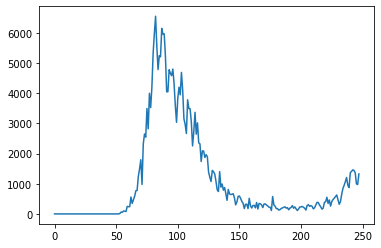While this plot shows the overall trend, it's hard to tell where the peak occurred, as there are no dates on the X-axis. We can use the date column as the index for the data frame to address this issue.

result_df.set_index('date', inplace=True)
result_df

Notice that the index of a data frame doesn't have to be numeric. Using the date as the index also allows us to get the data for a specific data using .loc.

result_df.loc['2020-09-01']
new_cases             9.960000e+02
total_cases           2.696595e+05
new_deaths            6.000000e+00
total_deaths          3.548300e+04
new_tests             5.439500e+04
total_tests           5.214766e+06
cases_per_million     4.459996e+03
deaths_per_million    5.868661e+02
tests_per_million     8.624890e+04
Name: 2020-09-01 00:00:00, dtype: float64

Let's plot the new cases & new deaths per day as line graphs.

result_df.new_cases.plot()
result_df.new_deaths.plot();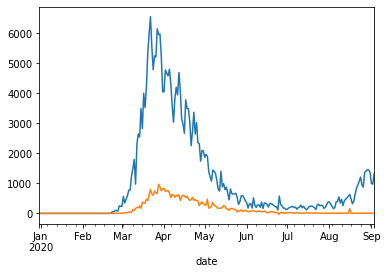We can also compare the total cases vs. total deaths.

result_df.total_cases.plot()
result_df.total_deaths.plot();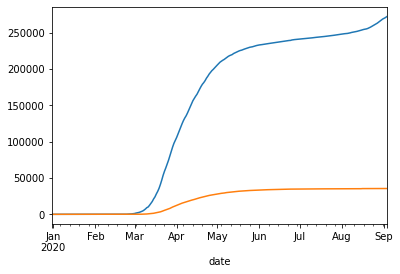Let's see how the death rate and positive testing rates vary over time.

death_rate = result_df.total_deaths / result_df.total_cases
death_rate.plot(title='Death Rate');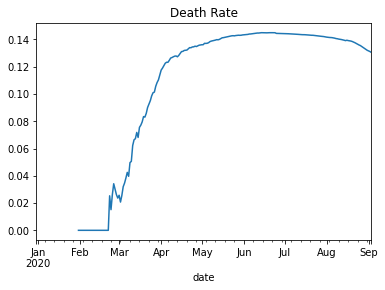positive_rates = result_df.total_cases / result_df.total_tests
positive_rates.plot(title='Positive Rate');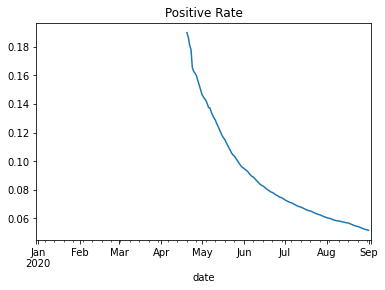Finally, let's plot some month-wise data using a bar chart to visualize the trend at a higher level.

covid_month_df.new_cases.plot(kind='bar');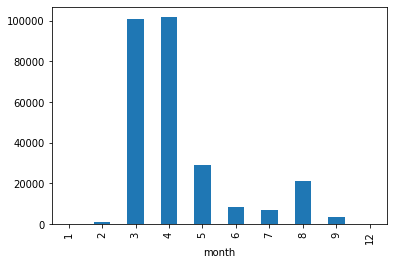covid_month_df.new_tests.plot(kind='bar')
<AxesSubplot:xlabel='month'>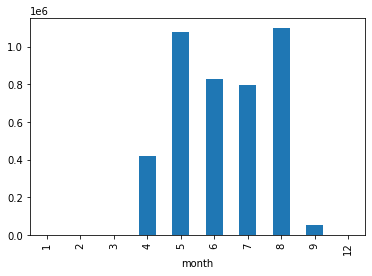Let's save and commit our work to Jovian.

import jovian
jovian.commit()
[jovian] Attempting to save notebook..

Exercises

Try the following exercises to become familiar with Pandas dataframe and practice your skills:

We've covered the following topics in this tutorial:

• Reading a CSV file into a Pandas data frame
• Retrieving data from Pandas data frames
• Querying, soring, and analyzing data
• Merging, grouping, and aggregation of data
• Extracting useful information from dates
• Basic plotting using line and bar charts
• Writing data frames to CSV files

You are ready to move on to the next tutorial: Data Visualization using Matplotlib & Seaborn.

Questions for Revision

Try answering the following questions to test your understanding of the topics covered in this notebook:

1. What is Pandas? What makes it useful?
2. How do you install the Pandas library?
3. How do you import the pandas module?
4. What is the common alias used while importing the pandas module?
5. How do you read a CSV file using Pandas? Give an example?
6. What are some other file formats you can read using Pandas? Illustrate with examples.
7. What are Pandas dataframes?
8. How are Pandas dataframes different from Numpy arrays?
9. How do you find the number of rows and columns in a dataframe?
10. How do you get the list of columns in a dataframe?
11. What is the purpose of the describe method of a dataframe?
12. How are the info and describe dataframe methods different?
13. Is a Pandas dataframe conceptually similar to a list of dictionaries or a dictionary of lists? Explain with an example.
14. What is a Pandas Series? How is it different from a Numpy array?
15. How do you access a column from a dataframe?
16. How do you access a row from a dataframe?
17. How do you access an element at a specific row & column of a dataframe?
18. How do you create a subset of a dataframe with a specific set of columns?
19. How do you create a subset of a dataframe with a specific range of rows?
20. Does changing a value within a dataframe affect other dataframes created using a subset of the rows or columns? Why is it so?
21. How do you create a copy of a dataframe?
22. Why should you avoid creating too many copies of a dataframe?
23. How do you view the first few rows of a dataframe?
24. How do you view the last few rows of a dataframe?
25. How do you view a random selection of rows of a dataframe?
26. What is the "index" in a dataframe? How is it useful?
27. What does a NaN value in a Pandas dataframe represent?
28. How is Nan different from 0?
29. How do you identify the first non-empty row in a Pandas series or column?
30. What is the difference between df.loc and df.at?
31. Where can you find a full list of methods supported by Pandas DataFrame and Series objects?
32. How do you find the sum of numbers in a column of dataframe?
33. How do you find the mean of numbers in a column of a dataframe?
34. How do you find the number of non-empty numbers in a column of a dataframe?
35. What is the result obtained by using a Pandas column in a boolean expression? Illustrate with an example.
36. How do you select a subset of rows where a specific column's value meets a given condition? Illustrate with an example.
37. What is the result of the expression df[df.new_cases > 100] ?
38. How do you display all the rows of a pandas dataframe in a Jupyter cell output?
39. What is the result obtained when you perform an arithmetic operation between two columns of a dataframe? Illustrate with an example.
40. How do you add a new column to a dataframe by combining values from two existing columns? Illustrate with an example.
41. How do you remove a column from a dataframe? Illustrate with an example.
42. What is the purpose of the inplace argument in dataframe methods?
43. How do you sort the rows of a dataframe based on the values in a particular column?
44. How do you sort a pandas dataframe using values from multiple columns?
45. How do you specify whether to sort by ascending or descending order while sorting a Pandas dataframe?
46. How do you change a specific value within a dataframe?
47. How do you convert a dataframe column to the datetime data type?
48. What are the benefits of using the datetime data type instead of object?
49. How do you extract different parts of a date column like the month, year, month, weekday, etc., into separate columns? Illustrate with an example.
50. How do you aggregate multiple columns of a dataframe together?
51. What is the purpose of the groupby method of a dataframe? Illustrate with an example.
52. What are the different ways in which you can aggregate the groups created by groupby?
53. What do you mean by a running or cumulative sum?
54. How do you create a new column containing the running or cumulative sum of another column?
55. What are other cumulative measures supported by Pandas dataframes?
56. What does it mean to merge two dataframes? Give an example.
57. How do you specify the columns that should be used for merging two dataframes?
58. How do you write data from a Pandas dataframe into a CSV file? Give an example.
59. What are some other file formats you can write to from a Pandas dataframe? Illustrate with examples.
60. How do you create a line plot showing the values within a column of dataframe?
61. How do you convert a column of a dataframe into its index?
62. Can the index of a dataframe be non-numeric?
63. What are the benefits of using a non-numeric dataframe? Illustrate with an example.
64. How you create a bar plot showing the values within a column of a dataframe?
65. What are some other types of plots supported by Pandas dataframes and series?